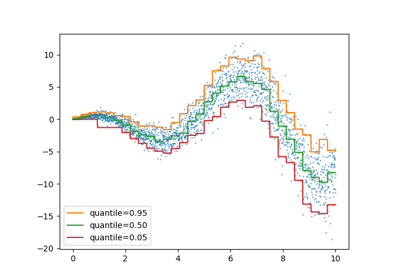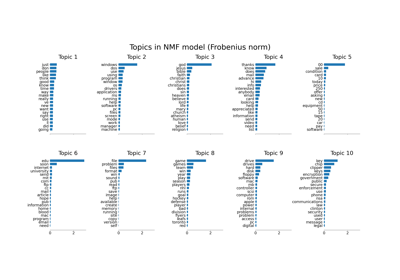# sklearn.decomposition.MiniBatchNMF¶

class sklearn.decomposition.MiniBatchNMF(n_components=None, *, init=None, batch_size=1024, beta_loss='frobenius', tol=0.0001, max_no_improvement=10, max_iter=200, alpha_W=0.0, alpha_H='same', l1_ratio=0.0, forget_factor=0.7, fresh_restarts=False, fresh_restarts_max_iter=30, transform_max_iter=None, random_state=None, verbose=0)[source]

Mini-Batch Non-Negative Matrix Factorization (NMF).

New in version 1.1.

Find two non-negative matrices, i.e. matrices with all non-negative elements, (W, H) whose product approximates the non-negative matrix X. This factorization can be used for example for dimensionality reduction, source separation or topic extraction.

The objective function is:

\begin{align}\begin{aligned}L(W, H) &= 0.5 * ||X - WH||_{loss}^2\\&+ alpha\_W * l1\_ratio * n\_features * ||vec(W)||_1\\&+ alpha\_H * l1\_ratio * n\_samples * ||vec(H)||_1\\&+ 0.5 * alpha\_W * (1 - l1\_ratio) * n\_features * ||W||_{Fro}^2\\&+ 0.5 * alpha\_H * (1 - l1\_ratio) * n\_samples * ||H||_{Fro}^2\end{aligned}\end{align}

Where:

$$||A||_{Fro}^2 = \sum_{i,j} A_{ij}^2$$ (Frobenius norm)

$$||vec(A)||_1 = \sum_{i,j} abs(A_{ij})$$ (Elementwise L1 norm)

The generic norm $$||X - WH||_{loss}^2$$ may represent the Frobenius norm or another supported beta-divergence loss. The choice between options is controlled by the beta_loss parameter.

The objective function is minimized with an alternating minimization of W and H.

Note that the transformed data is named W and the components matrix is named H. In the NMF literature, the naming convention is usually the opposite since the data matrix X is transposed.

Read more in the User Guide.

Parameters:
n_componentsint, default=None

Number of components, if n_components is not set all features are kept.

init{‘random’, ‘nndsvd’, ‘nndsvda’, ‘nndsvdar’, ‘custom’}, default=None

Method used to initialize the procedure. Valid options:

• None: ‘nndsvda’ if n_components <= min(n_samples, n_features), otherwise random.

• 'random': non-negative random matrices, scaled with: sqrt(X.mean() / n_components)

• 'nndsvd': Nonnegative Double Singular Value Decomposition (NNDSVD) initialization (better for sparseness).

• 'nndsvda': NNDSVD with zeros filled with the average of X (better when sparsity is not desired).

• 'nndsvdar' NNDSVD with zeros filled with small random values (generally faster, less accurate alternative to NNDSVDa for when sparsity is not desired).

• 'custom': use custom matrices W and H

batch_sizeint, default=1024

Number of samples in each mini-batch. Large batch sizes give better long-term convergence at the cost of a slower start.

beta_lossfloat or {‘frobenius’, ‘kullback-leibler’, ‘itakura-saito’}, default=’frobenius’

Beta divergence to be minimized, measuring the distance between X and the dot product WH. Note that values different from ‘frobenius’ (or 2) and ‘kullback-leibler’ (or 1) lead to significantly slower fits. Note that for beta_loss <= 0 (or ‘itakura-saito’), the input matrix X cannot contain zeros.

tolfloat, default=1e-4

Control early stopping based on the norm of the differences in H between 2 steps. To disable early stopping based on changes in H, set tol to 0.0.

max_no_improvementint, default=10

Control early stopping based on the consecutive number of mini batches that does not yield an improvement on the smoothed cost function. To disable convergence detection based on cost function, set max_no_improvement to None.

max_iterint, default=200

Maximum number of iterations over the complete dataset before timing out.

alpha_Wfloat, default=0.0

Constant that multiplies the regularization terms of W. Set it to zero (default) to have no regularization on W.

alpha_Hfloat or “same”, default=”same”

Constant that multiplies the regularization terms of H. Set it to zero to have no regularization on H. If “same” (default), it takes the same value as alpha_W.

l1_ratiofloat, default=0.0

The regularization mixing parameter, with 0 <= l1_ratio <= 1. For l1_ratio = 0 the penalty is an elementwise L2 penalty (aka Frobenius Norm). For l1_ratio = 1 it is an elementwise L1 penalty. For 0 < l1_ratio < 1, the penalty is a combination of L1 and L2.

forget_factorfloat, default=0.7

Amount of rescaling of past information. Its value could be 1 with finite datasets. Choosing values < 1 is recommended with online learning as more recent batches will weight more than past batches.

fresh_restartsbool, default=False

Whether to completely solve for W at each step. Doing fresh restarts will likely lead to a better solution for a same number of iterations but it is much slower.

fresh_restarts_max_iterint, default=30

Maximum number of iterations when solving for W at each step. Only used when doing fresh restarts. These iterations may be stopped early based on a small change of W controlled by tol.

transform_max_iterint, default=None

Maximum number of iterations when solving for W at transform time. If None, it defaults to max_iter.

random_stateint, RandomState instance or None, default=None

Used for initialisation (when init == ‘nndsvdar’ or ‘random’), and in Coordinate Descent. Pass an int for reproducible results across multiple function calls. See Glossary.

verbosebool, default=False

Whether to be verbose.

Attributes:
components_ndarray of shape (n_components, n_features)

Factorization matrix, sometimes called ‘dictionary’.

n_components_int

The number of components. It is same as the n_components parameter if it was given. Otherwise, it will be same as the number of features.

reconstruction_err_float

Frobenius norm of the matrix difference, or beta-divergence, between the training data X and the reconstructed data WH from the fitted model.

n_iter_int

Actual number of started iterations over the whole dataset.

n_steps_int

Number of mini-batches processed.

n_features_in_int

Number of features seen during fit.

feature_names_in_ndarray of shape (n_features_in_,)

Names of features seen during fit. Defined only when X has feature names that are all strings.

NMF

Non-negative matrix factorization.

MiniBatchDictionaryLearning

Finds a dictionary that can best be used to represent data using a sparse code.

References



“Fast local algorithms for large scale nonnegative matrix and tensor factorizations” Cichocki, Andrzej, and P. H. A. N. Anh-Huy. IEICE transactions on fundamentals of electronics, communications and computer sciences 92.3: 708-721, 2009.



“Algorithms for nonnegative matrix factorization with the beta-divergence” Fevotte, C., & Idier, J. (2011). Neural Computation, 23(9).

Examples

>>> import numpy as np
>>> X = np.array([[1, 1], [2, 1], [3, 1.2], [4, 1], [5, 0.8], [6, 1]])
>>> from sklearn.decomposition import MiniBatchNMF
>>> model = MiniBatchNMF(n_components=2, init='random', random_state=0)
>>> W = model.fit_transform(X)
>>> H = model.components_


Methods

 fit(X[, y]) Learn a NMF model for the data X. fit_transform(X[, y, W, H]) Learn a NMF model for the data X and returns the transformed data. get_feature_names_out([input_features]) Get output feature names for transformation. get_params([deep]) Get parameters for this estimator. Transform data back to its original space. partial_fit(X[, y, W, H]) Update the model using the data in X as a mini-batch. set_output(*[, transform]) Set output container. set_params(**params) Set the parameters of this estimator. Transform the data X according to the fitted MiniBatchNMF model.
fit(X, y=None, **params)[source]

Learn a NMF model for the data X.

Parameters:
X{array-like, sparse matrix} of shape (n_samples, n_features)

Training vector, where n_samples is the number of samples and n_features is the number of features.

yIgnored

Not used, present for API consistency by convention.

**paramskwargs

Parameters (keyword arguments) and values passed to the fit_transform instance.

Returns:
selfobject

Returns the instance itself.

fit_transform(X, y=None, W=None, H=None)[source]

Learn a NMF model for the data X and returns the transformed data.

This is more efficient than calling fit followed by transform.

Parameters:
X{array-like, sparse matrix} of shape (n_samples, n_features)

Data matrix to be decomposed.

yIgnored

Not used, present here for API consistency by convention.

Warray-like of shape (n_samples, n_components), default=None

If init='custom', it is used as initial guess for the solution.

Harray-like of shape (n_components, n_features), default=None

If init='custom', it is used as initial guess for the solution.

Returns:
Wndarray of shape (n_samples, n_components)

Transformed data.

get_feature_names_out(input_features=None)[source]

Get output feature names for transformation.

The feature names out will prefixed by the lowercased class name. For example, if the transformer outputs 3 features, then the feature names out are: ["class_name0", "class_name1", "class_name2"].

Parameters:
input_featuresarray-like of str or None, default=None

Only used to validate feature names with the names seen in fit.

Returns:
feature_names_outndarray of str objects

Transformed feature names.

get_params(deep=True)[source]

Get parameters for this estimator.

Parameters:
deepbool, default=True

If True, will return the parameters for this estimator and contained subobjects that are estimators.

Returns:
paramsdict

Parameter names mapped to their values.

inverse_transform(W)[source]

Transform data back to its original space.

New in version 0.18.

Parameters:
W{ndarray, sparse matrix} of shape (n_samples, n_components)

Transformed data matrix.

Returns:
X{ndarray, sparse matrix} of shape (n_samples, n_features)

Returns a data matrix of the original shape.

partial_fit(X, y=None, W=None, H=None)[source]

Update the model using the data in X as a mini-batch.

This method is expected to be called several times consecutively on different chunks of a dataset so as to implement out-of-core or online learning.

This is especially useful when the whole dataset is too big to fit in memory at once (see Strategies to scale computationally: bigger data).

Parameters:
X{array-like, sparse matrix} of shape (n_samples, n_features)

Data matrix to be decomposed.

yIgnored

Not used, present here for API consistency by convention.

Warray-like of shape (n_samples, n_components), default=None

If init='custom', it is used as initial guess for the solution. Only used for the first call to partial_fit.

Harray-like of shape (n_components, n_features), default=None

If init='custom', it is used as initial guess for the solution. Only used for the first call to partial_fit.

Returns:
self

Returns the instance itself.

set_output(*, transform=None)[source]

Set output container.

See Introducing the set_output API for an example on how to use the API.

Parameters:
transform{“default”, “pandas”}, default=None

Configure output of transform and fit_transform.

• "default": Default output format of a transformer

• "pandas": DataFrame output

• None: Transform configuration is unchanged

Returns:
selfestimator instance

Estimator instance.

set_params(**params)[source]

Set the parameters of this estimator.

The method works on simple estimators as well as on nested objects (such as Pipeline). The latter have parameters of the form <component>__<parameter> so that it’s possible to update each component of a nested object.

Parameters:
**paramsdict

Estimator parameters.

Returns:
selfestimator instance

Estimator instance.

transform(X)[source]

Transform the data X according to the fitted MiniBatchNMF model.

Parameters:
X{array-like, sparse matrix} of shape (n_samples, n_features)

Data matrix to be transformed by the model.

Returns:
Wndarray of shape (n_samples, n_components)

Transformed data.

## Examples using sklearn.decomposition.MiniBatchNMF¶Release Highlights for scikit-learn 1.1

Release Highlights for scikit-learn 1.1Topic extraction with Non-negative Matrix Factorization and Latent Dirichlet Allocation

Topic extraction with Non-negative Matrix Factorization and Latent Dirichlet Allocation